# The Measurement of Polymer Films

The MProbe can be used for the analysis of many different types of polymer films. Depending on the specific challenges of each application, a variety of configurations can be used:

1. The MProbe Vis system has a wavelength range between 400 and 1000 nm, and can be used to analyze polymer-based films with thicknesses between 15 nm and 20 µm. For films with a thickness above 500 nm, the thickness and the films refractive index can be determined. For thinner films, in most cases, only the thickness can be determined.

2. The MProbeVisHR is designed for the measurement of polymer films, which are either colored or have scattering, and with a thickness of greater than 20 μm.

3. The MProbe UVVis has a wavelength range between 200 and 1000 nm and is used in applications where the optical properties of a polymer must be known. The majority of polymers absorb in the UV, allowing the optical parameters (n and k) and the thickness to be determined. The MProbe UVVis can also be used to determine the thickness of very thin polymer monolayers.

## I. Thickness and Refractive Index (R.I.) Measurement (MProbe Vis and MProbe VisHR)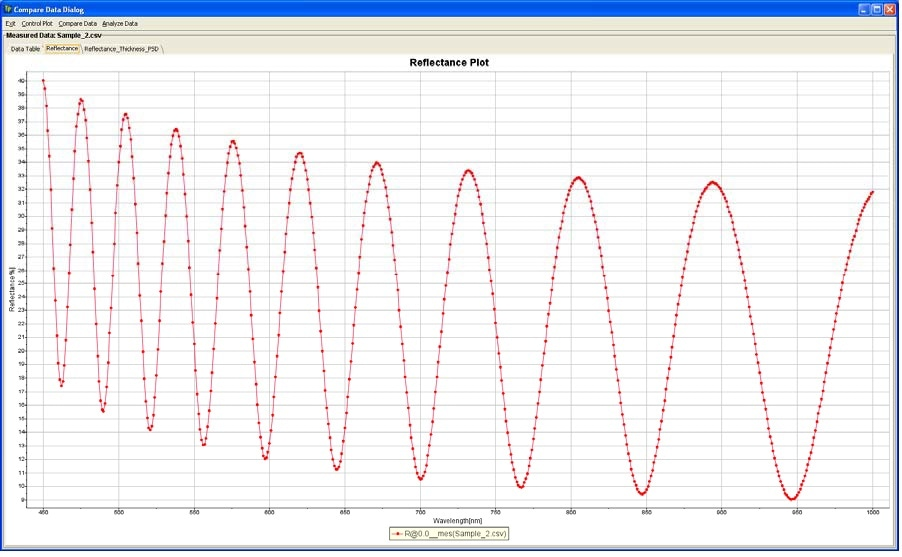Figure 1. Reflectance spectrum of the polymer film on Si (MProbe Vis)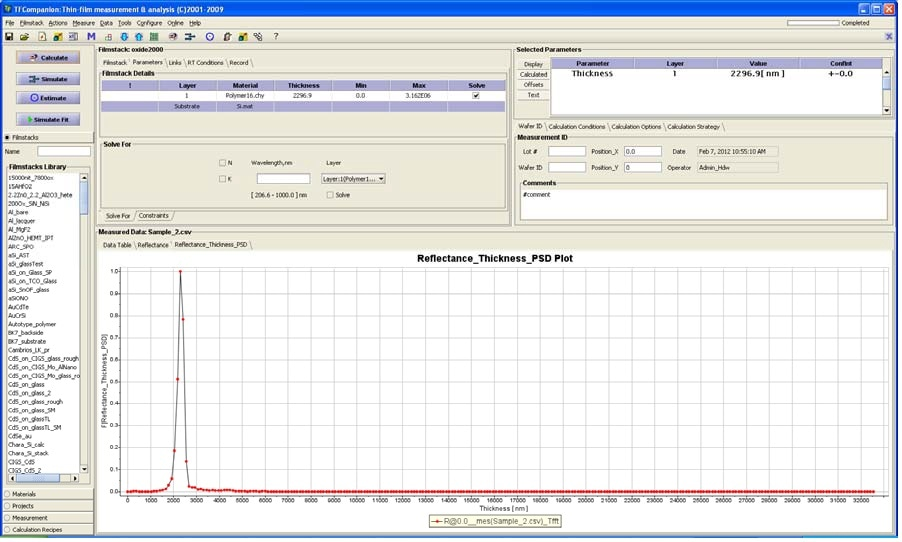Figure 2. Using a “thick film algorithm” to estimate the thickness of the films (standard polymer material R.I.~ 1.6 is used)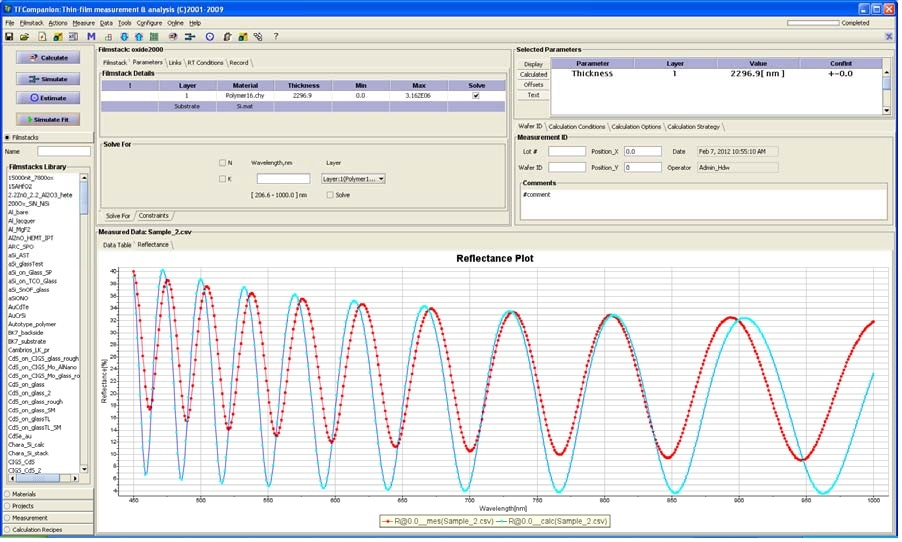Figure 3. Using direct fit shows that R.I is not correct – it should be adjusted to have a good fit between measured data and the model. The amplitude of the oscillations is a measure of optical contrast (refractive index difference) between two materials of the interface.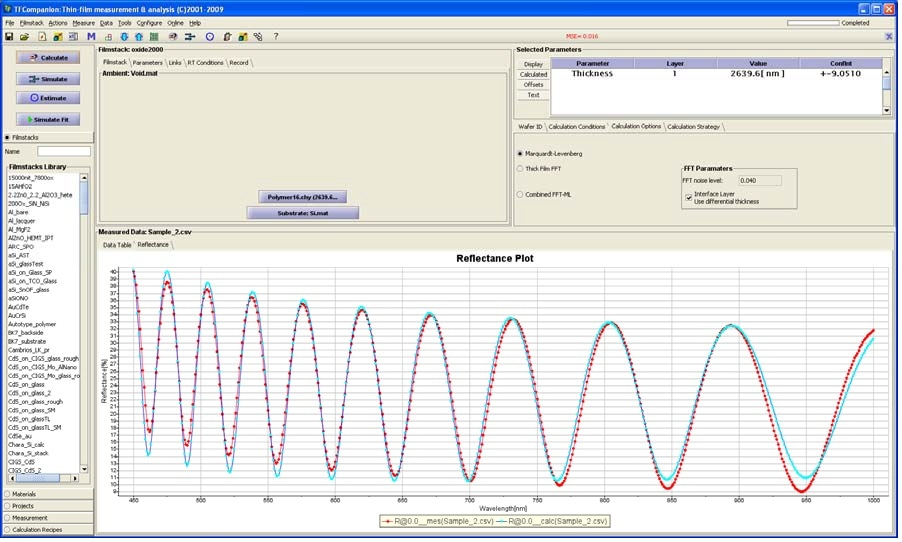Figure 4. Fit of thickness and R.I. gives better result.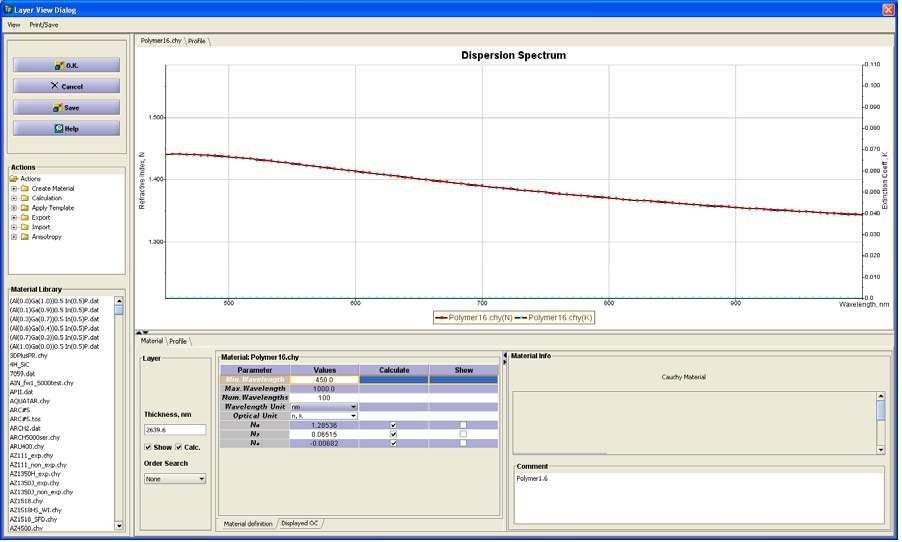Figure 5. R.I. spectrum determined from the measurement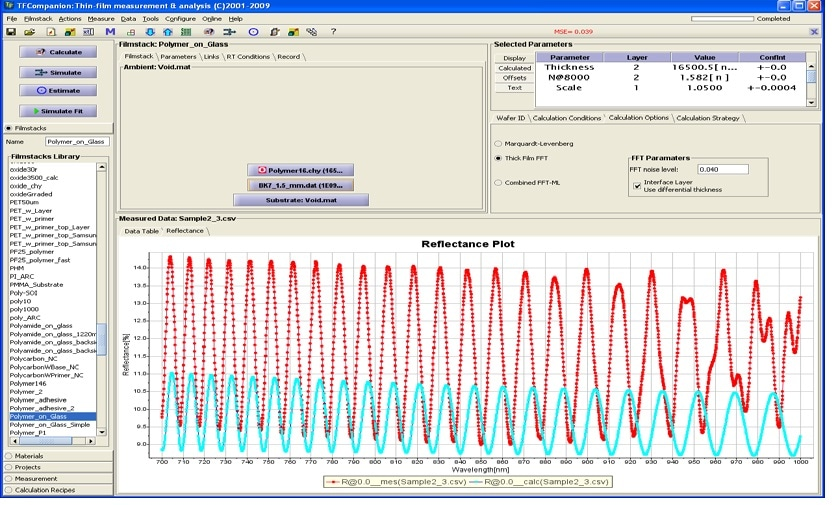Figure 6. Measurement of thick polymer on thin glass (1.3 mm) using MProbe VisHR.
Large mismatch between the measurement and the model shows that refractive index of the polymer is not correct. Optical contrast between glass and polymer is much higher i.e. polymer’ R.I. should be higher.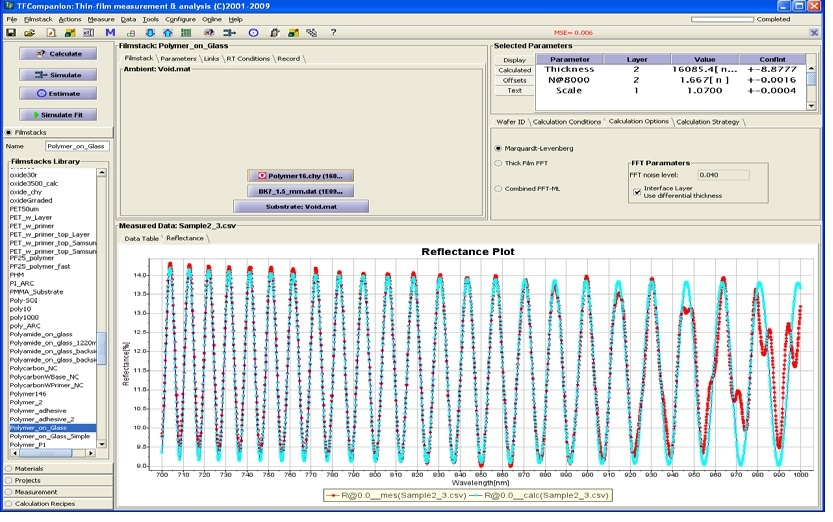Figure 7. Polymer refractive index is adjusted to fit the measured data.
The difference in R.I. between Figure 6 (R.I.=1.582) and Figure 7 (R.I.=1.667) is ~0.08
Precision of R.I.<0.001 (1 σ) can be achieved.

## II. Measurement of a Thick Polymer Films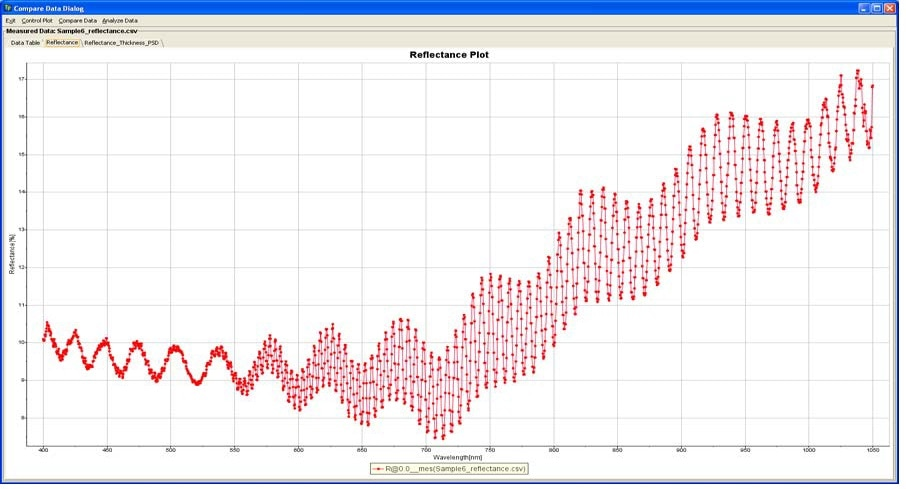Figure 8. Reflectance spectrum of the a free-standing polymer film with polymer coating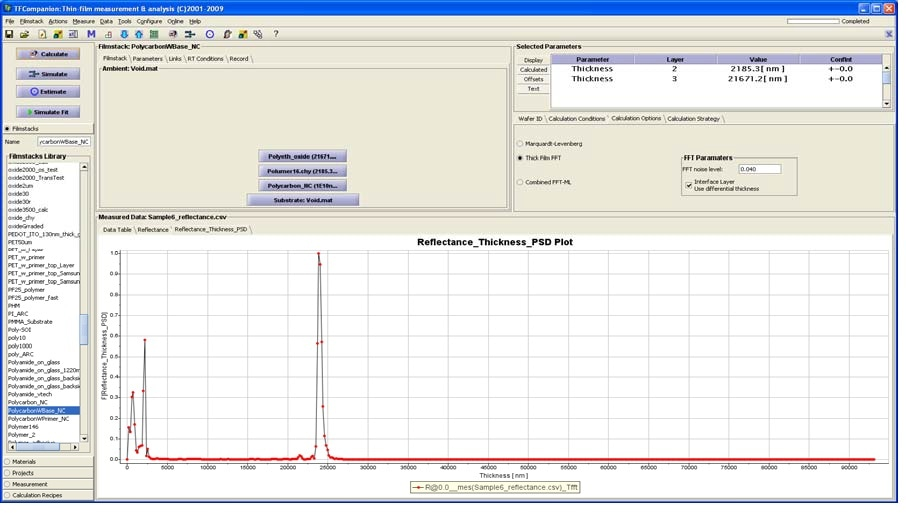Figure 9. Thicknesses of both layers can be easily determined using thick-film algorithm as 21.8 um and 2.1 um (thicknesses are indicated by position of the peaks)

## III. Measurement of Polymer Films of PET Substrate (Touch-Screen Application)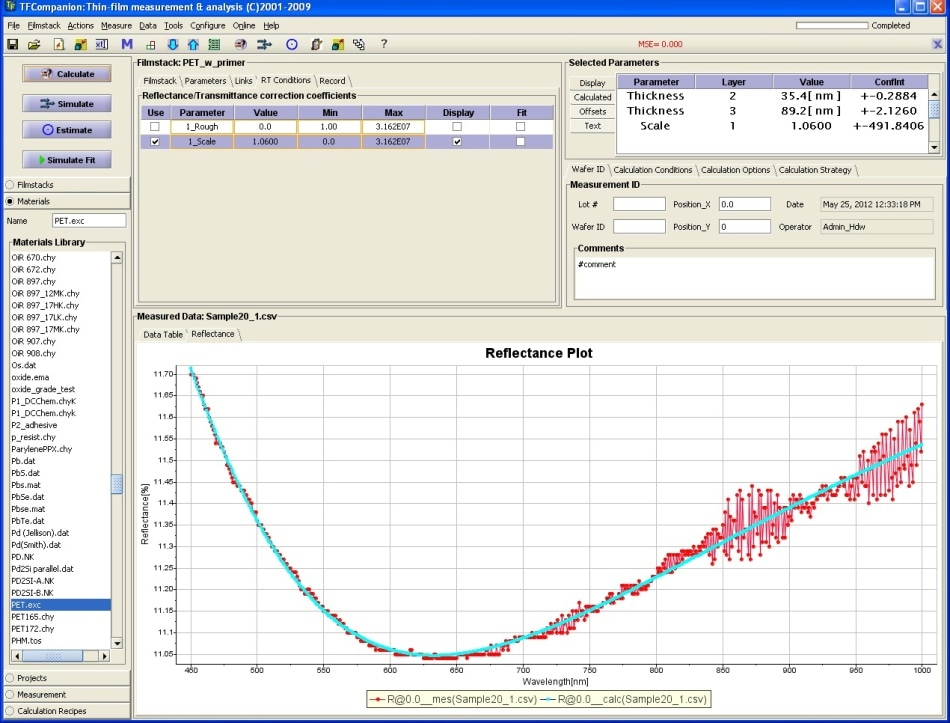Figure 10. Measurement of the PET sample with primer and main polymer layer (35 nm primer, 85 nm additional polymer layer). Model to measured data fit. The “noise” in spectrum due to the effect of the thin PET substrate. PET n,k were determined from measurement of the blank PET sample.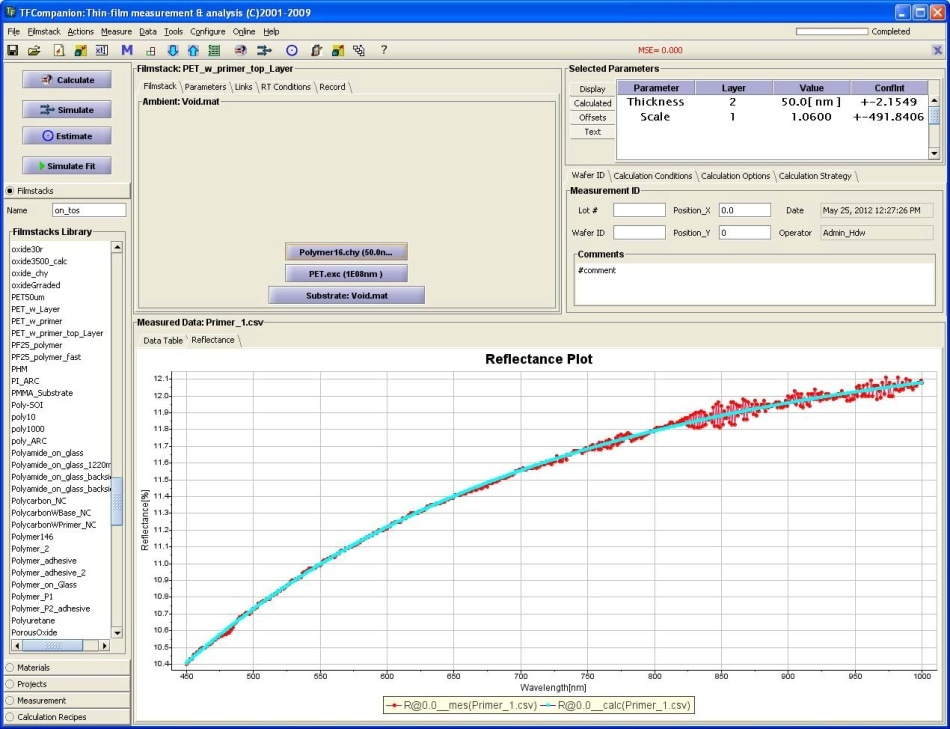Figure 11. Measurement of the PET sample with primer.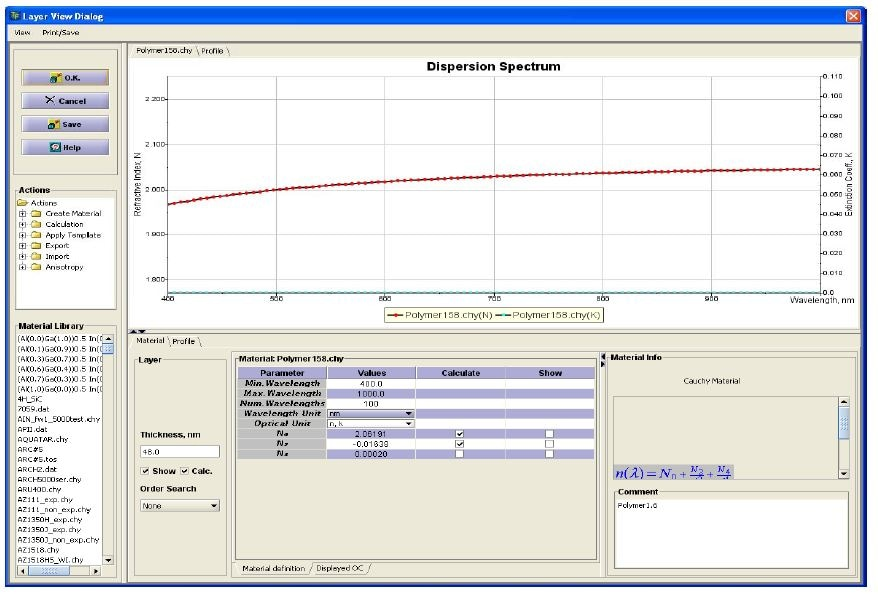Figure 12. Primer R.I spectrum determine from the measurement.

## IV. Measurement Polymers on Flexible Printed Board (MProbe VisHR)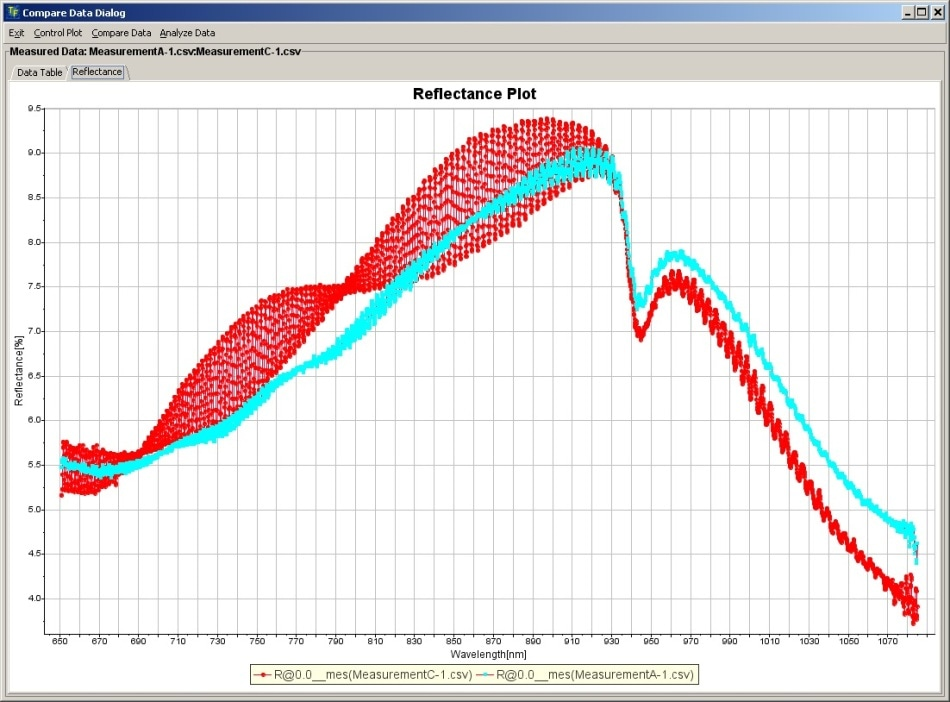Figure 13. Reflectance spectrum at 2 points on the printed board showing that at least 2 films are present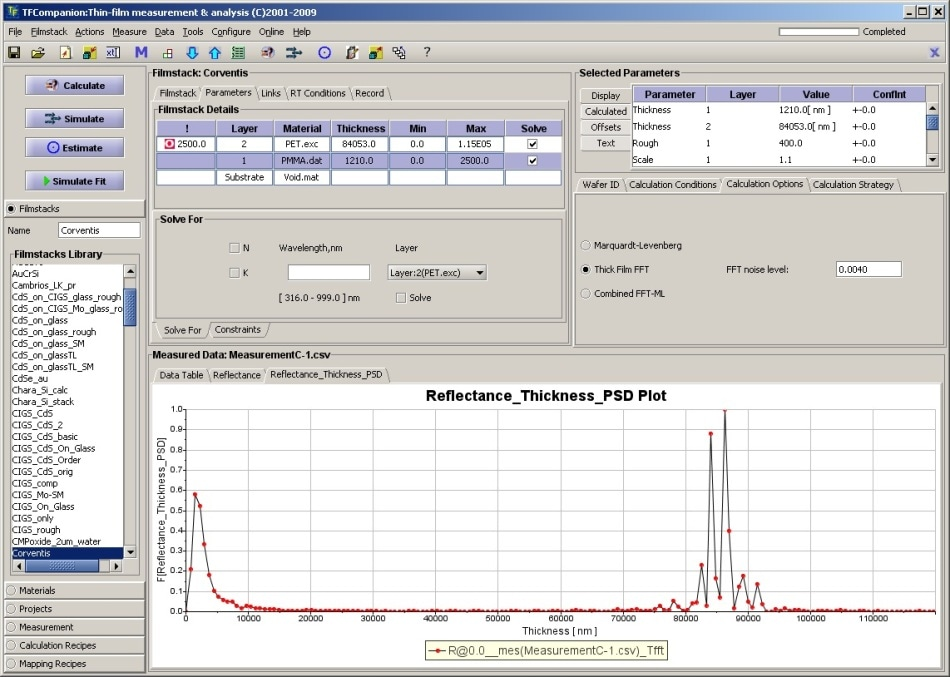Figure 14. Results of the spectrum analysis. 1.2 µm polymer film (peak 1), 84 µm PET (peak 2). Peak 3 is a total thickness PET+polymer (85.2 µm)

## V. Conductive Polymer Layer on PET (MProbe Vis)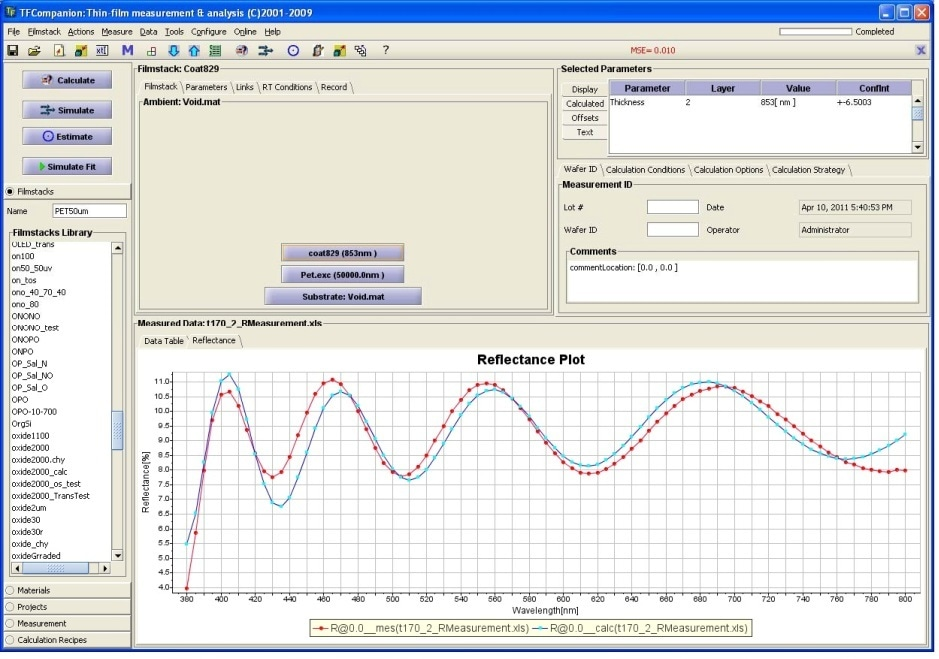Figure 15. Conductive polymer layer on PET substrate (Model vs. measurement). Thickness: 853 nm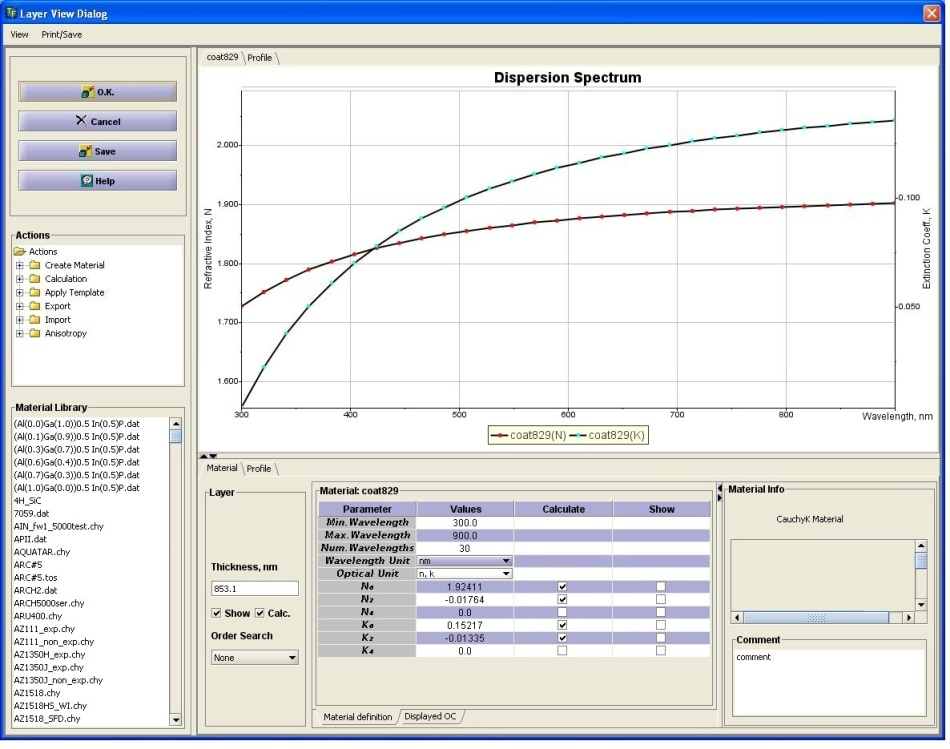Figure 16. Optical constants of conductive polymer determined form the measurement.

## VI. Measurement of Biopolymer Coating on Implant Stent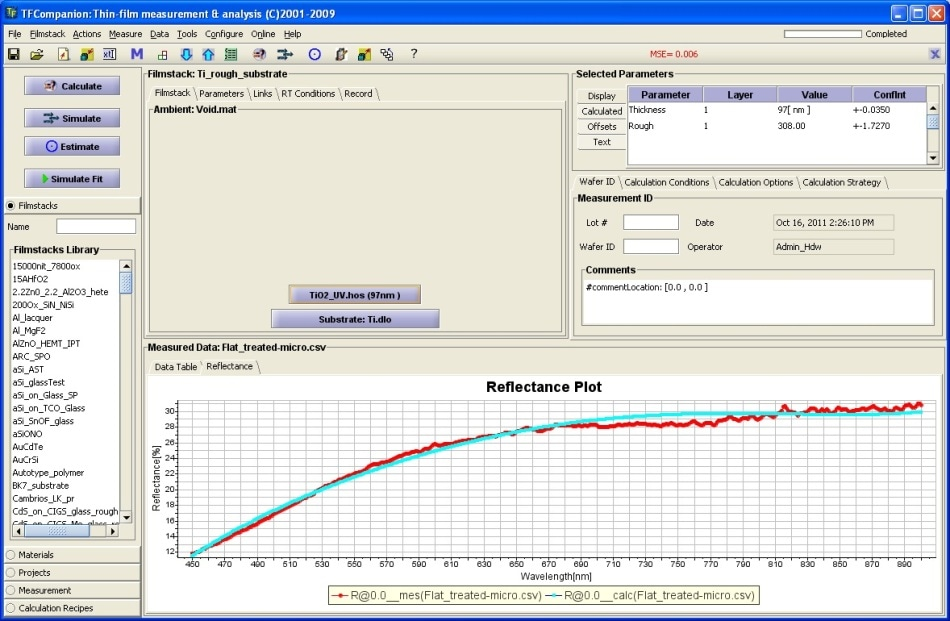Figure 17. Biopolymer film on Ti stent. Model vs. measurement. Film thickness: 97 nm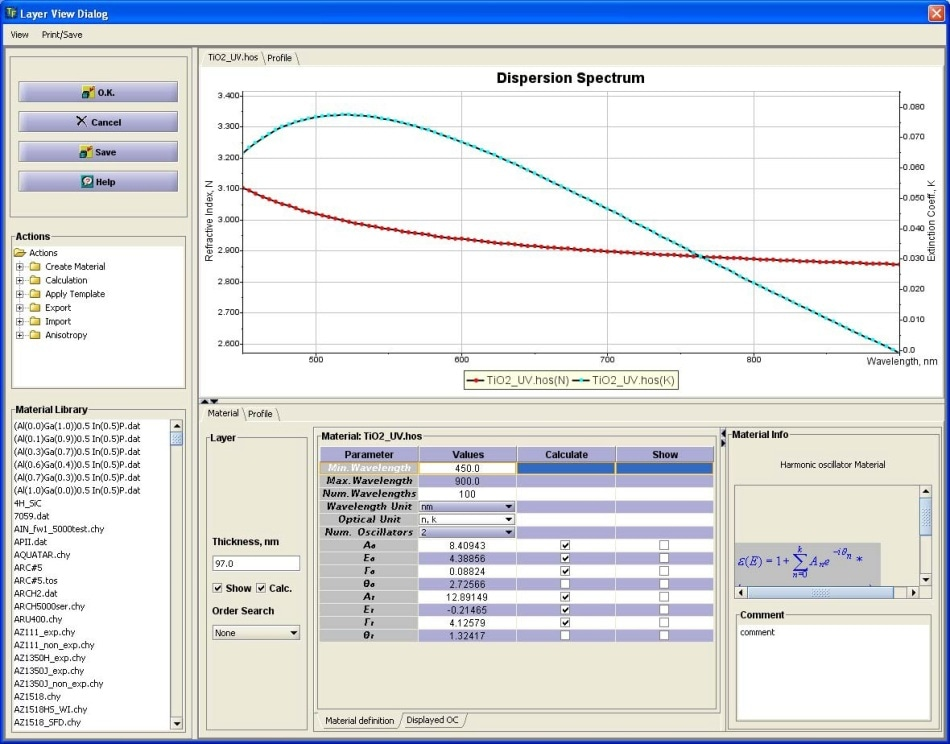Figure 18. Optical constants of the polymer film determined form the measurement.

## VII. Polymer Film Measurement - Optical Constants Analysis (MProbe UVVis)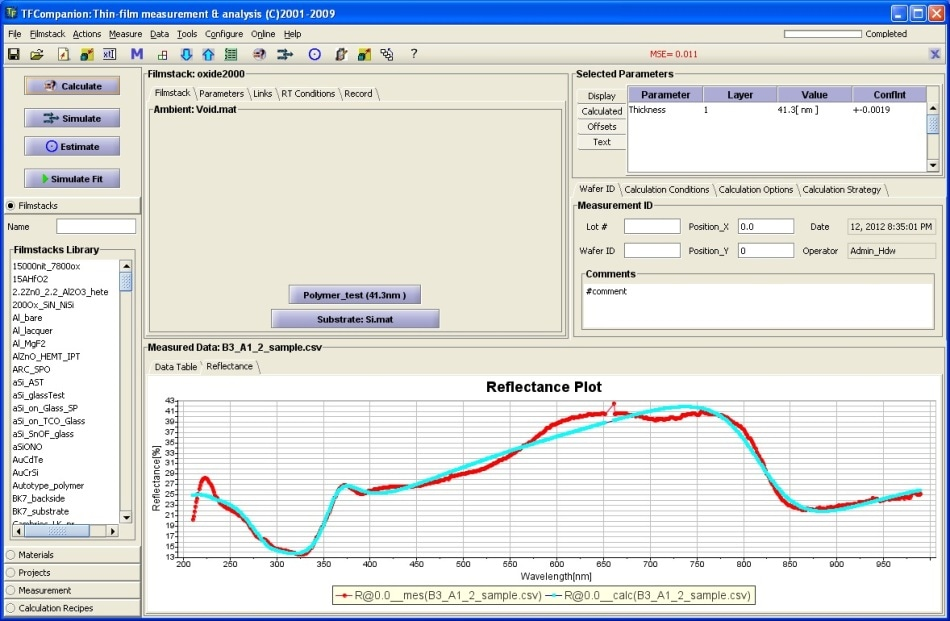Figure 19. Measurement of polymer with squaraine dye deposited on glass. Model vs. measured data. Thickness 41 nm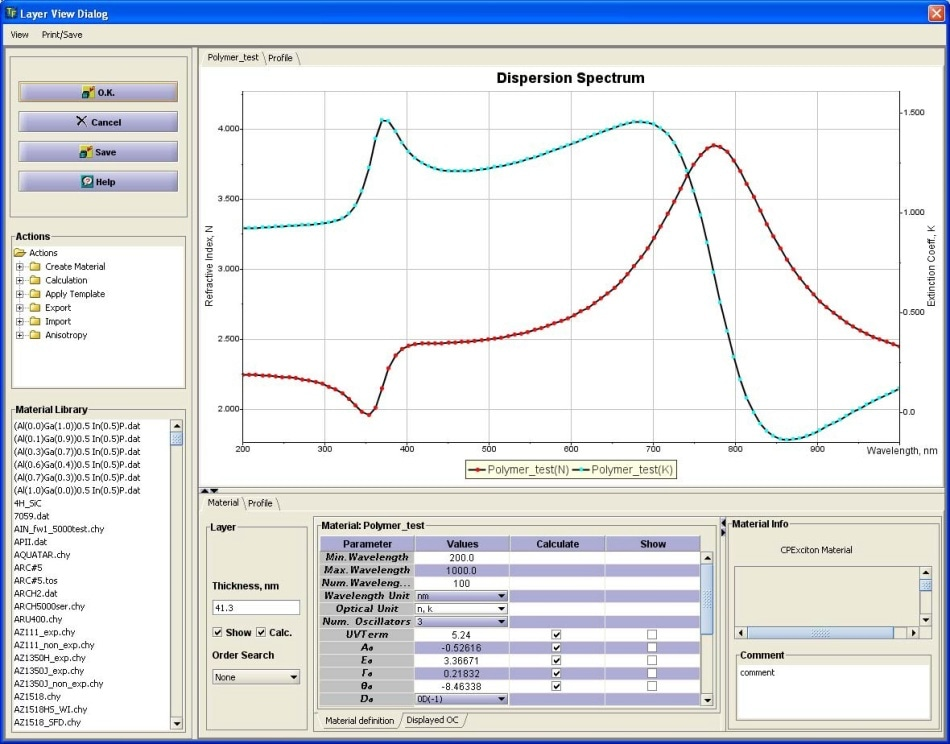Figure 20. Optical constants of the polymer determined from the measurement.

## VIII. Measurement of Thin Polymers (MProbe UVVis)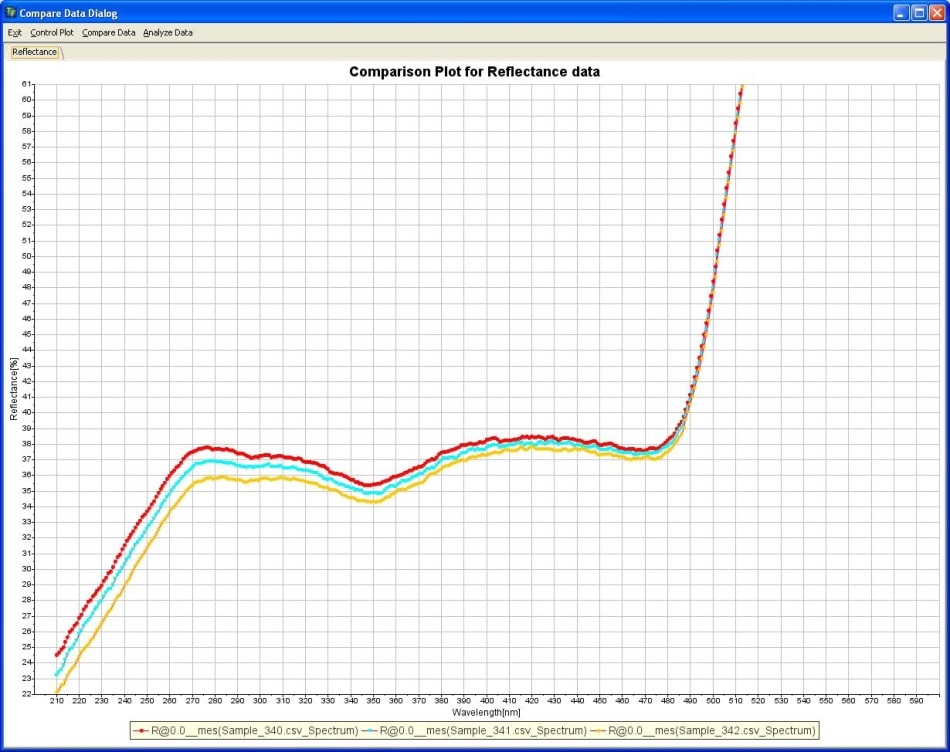Figure 21. Reflectance spectra of gold (uncoated), 1.3 nm polymer/gold, 2.6 nm polymer/ gold. Polymer monolayer can be easily distinguished and measured.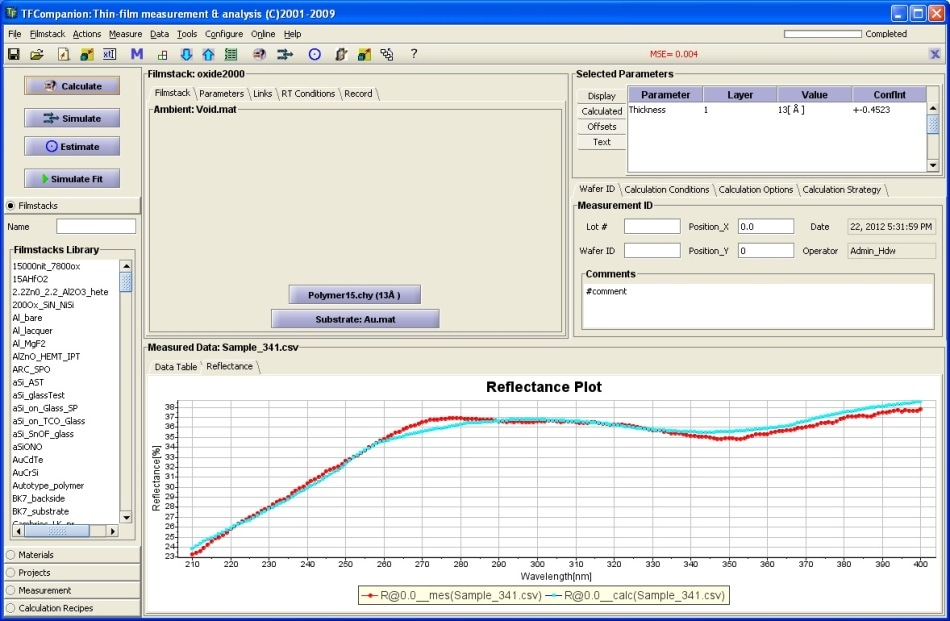Figure 22. Measurement of the polymer monolayer (1.3 nm). Model vs. measurement.This information has been sourced, reviewed and adapted from materials provided by SemiconSoft.

## Citations

• APA

SemiconSoft. (2018, October 25). The Measurement of Polymer Films. AZoM. Retrieved on November 28, 2020 from https://www.azom.com/article.aspx?ArticleID=16811.

• MLA

SemiconSoft. "The Measurement of Polymer Films". AZoM. 28 November 2020. <https://www.azom.com/article.aspx?ArticleID=16811>.

• Chicago

SemiconSoft. "The Measurement of Polymer Films". AZoM. https://www.azom.com/article.aspx?ArticleID=16811. (accessed November 28, 2020).

• Harvard

SemiconSoft. 2018. The Measurement of Polymer Films. AZoM, viewed 28 November 2020, https://www.azom.com/article.aspx?ArticleID=16811.

## Tell Us What You Think